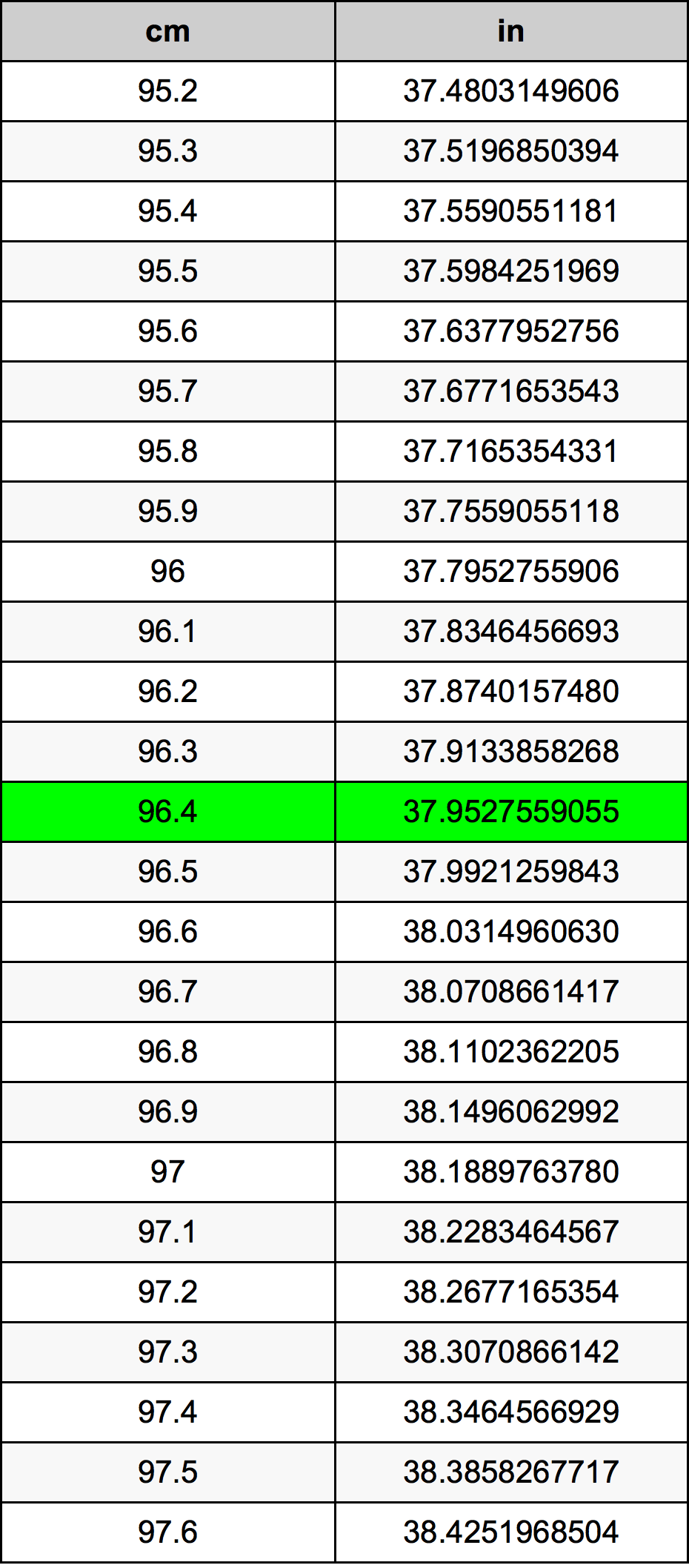Cm To Inches

# 96.4 cm to in96.4 Centimeters to Inches

cm
=
in

## How to convert 96.4 centimeters to inches?

 96.4 cm * 0.3937007874 in = 37.9527559055 in 1 cm
A common question is How many centimeter in 96.4 inch? And the answer is 244.856 cm in 96.4 in. Likewise the question how many inch in 96.4 centimeter has the answer of 37.9527559055 in in 96.4 cm.

## How much are 96.4 centimeters in inches?

96.4 centimeters equal 37.9527559055 inches (96.4cm = 37.9527559055in). Converting 96.4 cm to in is easy. Simply use our calculator above, or apply the formula to change the length 96.4 cm to in.

## Convert 96.4 cm to common lengths

UnitLength
Nanometer964000000.0 nm
Micrometer964000.0 µm
Millimeter964.0 mm
Centimeter96.4 cm
Inch37.9527559055 in
Foot3.1627296588 ft
Yard1.0542432196 yd
Meter0.964 m
Kilometer0.000964 km
Mile0.0005990018 mi
Nautical mile0.0005205184 nmi

## What is 96.4 centimeters in in?

To convert 96.4 cm to in multiply the length in centimeters by 0.3937007874. The 96.4 cm in in formula is [in] = 96.4 * 0.3937007874. Thus, for 96.4 centimeters in inch we get 37.9527559055 in.

## 96.4 Centimeter Conversion Table## Alternative spelling

96.4 cm to in, 96.4 cm in in, 96.4 Centimeters to in, 96.4 Centimeters in in, 96.4 Centimeter to in, 96.4 Centimeter in in, 96.4 Centimeters to Inch, 96.4 Centimeters in Inch, 96.4 Centimeter to Inch, 96.4 Centimeter in Inch, 96.4 cm to Inch, 96.4 cm in Inch, 96.4 Centimeter to Inches, 96.4 Centimeter in Inches